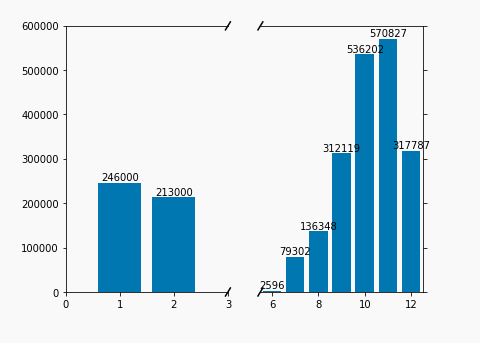# Creating Charts with Broken Axes

Sometimes outliers are just in the way of chart creation and we want to put them in their own section of chart. Python can help us do that! I started with a data set like "count of users created by month" where there were only values for Jan-Feb and June-Dec. I wanted to split these up rather than look at the blank middle of the year.  This was done by (1) creating two subplots, (2) plotting the same data on each, but then (3) limiting each x axis to the chosen range. We then (4) remove the inner edges of each subplot and (5) add the tick marks to indicate a broken axis. I then (6) added data labels for good measure. The code I used is below:

import pandas as pd
import matplotlib.pylab as plt
import numpy as np

z=np.array(df)
x= z[:,0]
y = z[:,1]
# 1. create two subplots
f,(ax,ax2) = plt.subplots(1,2,sharey=True, facecolor='w')

# 2. plot the same data on both axes
ax.bar(x, y)
ax2.bar(x, y)

# 3. limit each x axis to the chosen range
a=0
b=3
c=5.5
d=12.5
ax.set_xlim(a,b)
ax2.set_xlim(c,d)
# ax.set_xlim(0,3)
# ax2.set_xlim(5.5,12.5)

# 4. hide the spines between ax and ax2
ax.spines['right'].set_visible(False)
ax2.spines['left'].set_visible(False)
ax.yaxis.tick_left()
ax.tick_params(labelright='off')
ax2.yaxis.tick_right()

# 5. This looks pretty good, and was fairly painless, but you can get that
# cut-out diagonal lines look with just a bit more work. The important
# thing to know here is that in axes coordinates, which are always
# between 0-1, spine endpoints are at these locations (0,0), (0,1),
# (1,0), and (1,1).  Thus, we just need to put the diagonals in the
# appropriate corners of each of our axes, and so long as we use the
# right transform and disable clipping.

d = .015 # how big to make the diagonal lines in axes coordinates
# arguments to pass plot, just so we don't keep repeating them
kwargs = dict(transform=ax.transAxes, color='k', clip_on=False)
ax.plot((1-d,1+d), (-d,+d), **kwargs)
ax.plot((1-d,1+d),(1-d,1+d), **kwargs)

kwargs.update(transform=ax2.transAxes)  # switch to the bottom axes
ax2.plot((-d,+d), (1-d,1+d), **kwargs)
ax2.plot((-d,+d), (-d,+d), **kwargs)

# ax and ax2 via f.subplots_adjust(hspace=...) or plt.subplot_tool(),
# the diagonal lines will move accordingly, and stay right at the tips
# of the spines they are 'breaking'

# 6. Make some labels.
rects = ax.patches
labels = ["%d" % i for i in y]
for i, rect, label in zip(x,rects, labels):
height = rect.get_height()
print(i)
if i < b:
ax.text(rect.get_x() + rect.get_width() / 2, height + 5, label,
ha='center', va='bottom')
elif i > c:
ax2.text(rect.get_x() + rect.get_width() / 2, height + 5, label,
ha='center', va='bottom')

plt.show()
periscope.output(plt)Sub Plots can also be made with one on top of the other, so a similar thing can be done for cutting y Axes. Would you go about this in a different way? How did you do it? How does yours look?